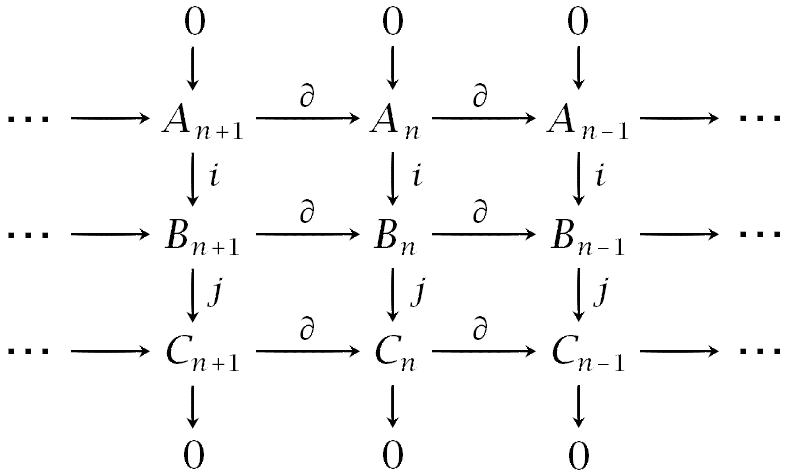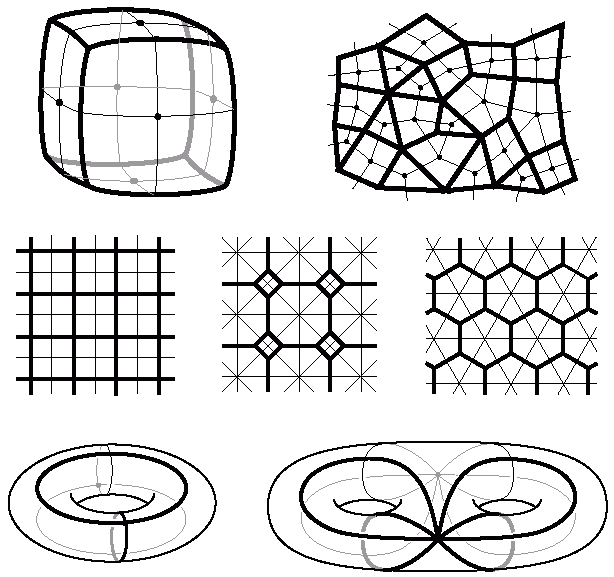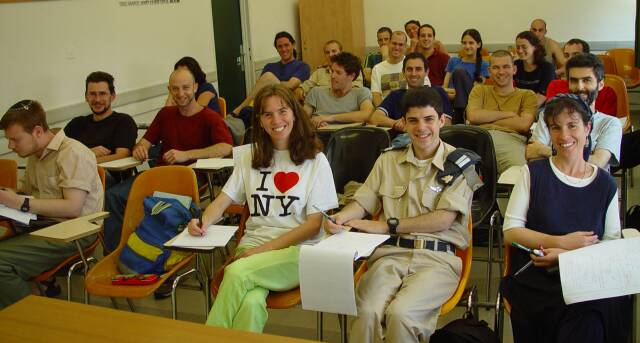Dror Bar-Natan: Classes: 2001-02:

# Fundamental Concepts in Algebraic Topology

Instructor: Dror Bar-Natan, drorbn@math.huji.ac.il, 02-658-4187.

Classes: Tuesdays 12:00-14:00 at Mathematics 110 and Thursdays 12:00-14:00 at Sprintzak 213.

Review sessions: Thursdays 14:00-15:00 with Boris Chorny chorny@math.huji.ac.il.

Office hours: Tuesdays 14:00-15:00 in my office, Mathematics 309.

Agenda: Learn how algebra and topology interact in the field of Algebraic Topology.

Syllabus: We will shamelessly follow Hatcher's book and cover the following topics: the fundamental group, Van Kampen's theorem, covering spaces, simplicial and singular homology, computations of homology, cohomology, cup products, Poincaré duality and more if we have the time.

Prerequisites: Point set topology and some basic notions of algebra - groups, rings, etc.

Reading material: (each student must have a copy)Weekly Material: (Also use the primitive Class Notes Browser)

 March 12, 14 Class notes for March 12th (the basic idea of algebraic topology, Brouwer's theorem, the fundamental group, the fundamental group of the circle). Homework assignment #1: Ex1.ps, Ex1.png (category theory, fundamental group calculations, an application of Brouwer's theorem). Class notes for March 14th (the lifting property for covering spaces, the fundamental theorem of algebra, Brouwer's fixed point theorem) March 19, 21 Class notes for March 19th (simple properties of the fundamental group, free products of groups, formulation of Van-Kampen's theorem). Homework assignment #2: Ex2.ps, Ex2-1.png, Ex2-2.png (Van-Kampen's theorem, invariance of the Euler characteristic) Class notes for March 21st (Van-Kampen's theorem - examples and proof) March 26, 28 Passover vacation April 2, 4 Passover vacation April 9, 11 Class notes for April 9th (Emmanuel Farjoun on further Van-Kampen examples - Dror was sick). Homework assignment #3: Ex3.ps, Ex3.png (Fundamental groups of knot complements, covering maps). Class notes for April 11th (end of the Van-Kampen proof, basics of covering spaces). April 16, 18 April 16 is Memorial Day Handout on Covering Spaces. Homework assignment #4: Ex4.ps, Ex4.png (more on covering spaces). Class notes for April 18th (the lifting property, the universal covering space). April 23, 25 Handout on The Universal Covering. Class notes for April 23rd (Construction of the universal cover, a unique covering for every group). "Last Class on Covering Spaces" handout: CoveringsLastClass.pdf, CoveringsLastClass.jpg. Class notes for April 25th ("Last class on covering spaces"). Homework assignment #5: Ex5.ps, Ex5.png (more on covering spaces). April 30, May 2 Handout on "Covering Spaces Done Right": CoveringsDoneRight.pdf, CoveringsDoneRight.ps. Class notes for April 30th (a functor from the category of covering spaces to the category of G-sets). Homework assignment #6: Ex6.ps, Ex6.png (the fundamental group of SO(3)). May 7, 9 Class notes for May 7th (the intuition of homology, precise definition, the square of the boundary map, the homology of a point). Class notes for May 9th (homology is a functor, homotopy invariance of homology). Homework assignment #7: Ex7.ps, Ex7-1.png, Ex7-2.png (diagram chasing). May 14, 16 May 16 is Shavuot May 21, 23 May 21 is Student's Day. Homework assignment #8: Ex8.ps, Ex8.png (more diagram chasing, singular homology computation). Class notes for May 23rd (the long exact sequence for a quotient space, assuming excision). May 28, 30 Handout on the barycentric subdivision: BarycentricSubdivision.gif. Class notes for May 28th (proof of excision). Homework assignment #9: Ex9.ps, Ex9.png (the long exact sequence of a triple, some homology calculations). Class notes for May 30th (explicit generators, simplicial structures). June 4, 6 Class notes for June 4th (homology of simplicial structures, definition of degrees). Class notes for June 6th (theorem on degrees and local degrees, the linking number). Homework assignment #10: Ex10.ps, Ex10.png (simplicial homology, degrees). June 11, 13 Print your own Rayman-Excision stickers! Get RaymanExcision.png. Print your own Euler characteristic stickers! Get EulerCharacteristic.png. (Torus by Tom Longtin). Class notes for June 11th (review of degrees, CW complexes, the Euler characteristic). Homework assignment #11: Ex11.ps, Ex11.png (Euler characteristic, Mayer-Vietoris, CW-homology). June 18, 20 Handout on Topological Theorems About Rn (PDF). Handout on Topological Pathologies in R3. Class notes for June 18th (normalized simplices, theorems and pathologies in Euclidean spaces). Homework assignment #12: Ex12.ps, Ex12.png (classical applications). Class notes for June 20th (the homology of the comlement of a sphere in another one, applications to the linking number, invariance of domain). June 25, 27 Class notes for June 25th and 27th (end of invariance of domain, the Borsuk-Ulam theorem) July 7, 9, 10, 11 Review class: Sprintzak 217, July 7th at 10:00. See handout and sample test. Office hours: July 9 and 10, Math 309, 11:0-13:00. Final examination (Moed A), Rashall Auditorium, July 11th at 10:00-13:00: MoedA.pdf, MoedA.gif (solution to the riddle). August 28 Final examination (Moed B): Math 2, 10:00-13:00 (I was not present).

Class Photo, May 9, 2002Click on the image to get a bigger version

Illustrations from Hatcher's book, background image from http://www.sover.net/~tlongtin/puzzles/hexrndtor500.html.